# Equilibrium of Forces

In this lecture, we will study how to formulate the equation of equilibrium.

When multiple forces are acting on an object and the composed force of these forces is zero, we say that the forces are “equilibrated”.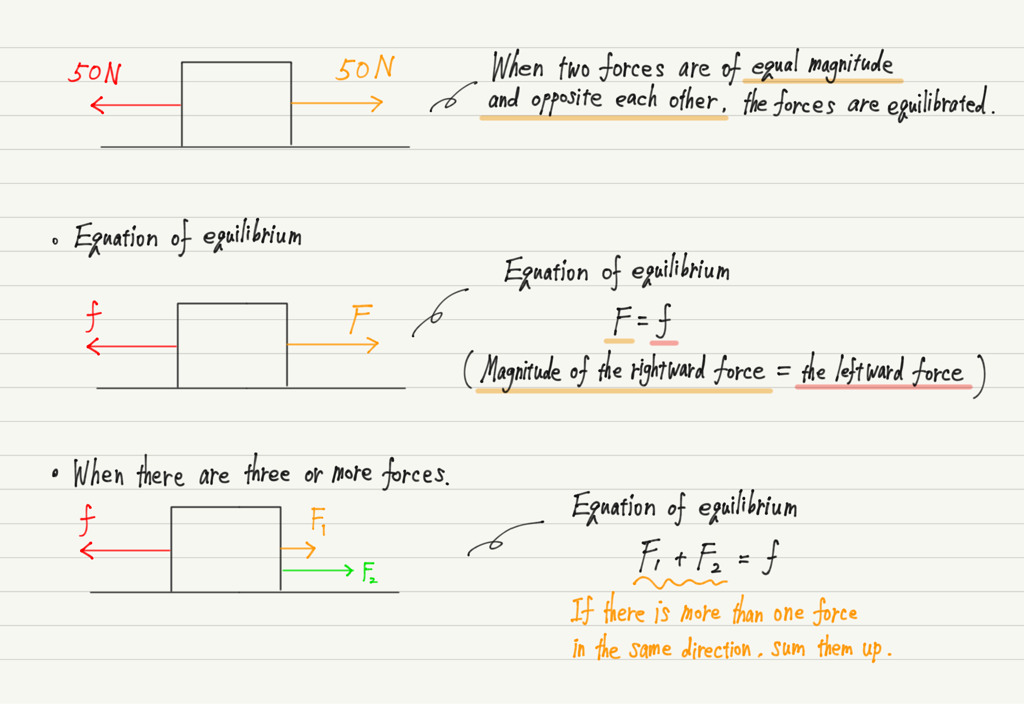## Calculation of the equilibrium of forces

Try the following exercise.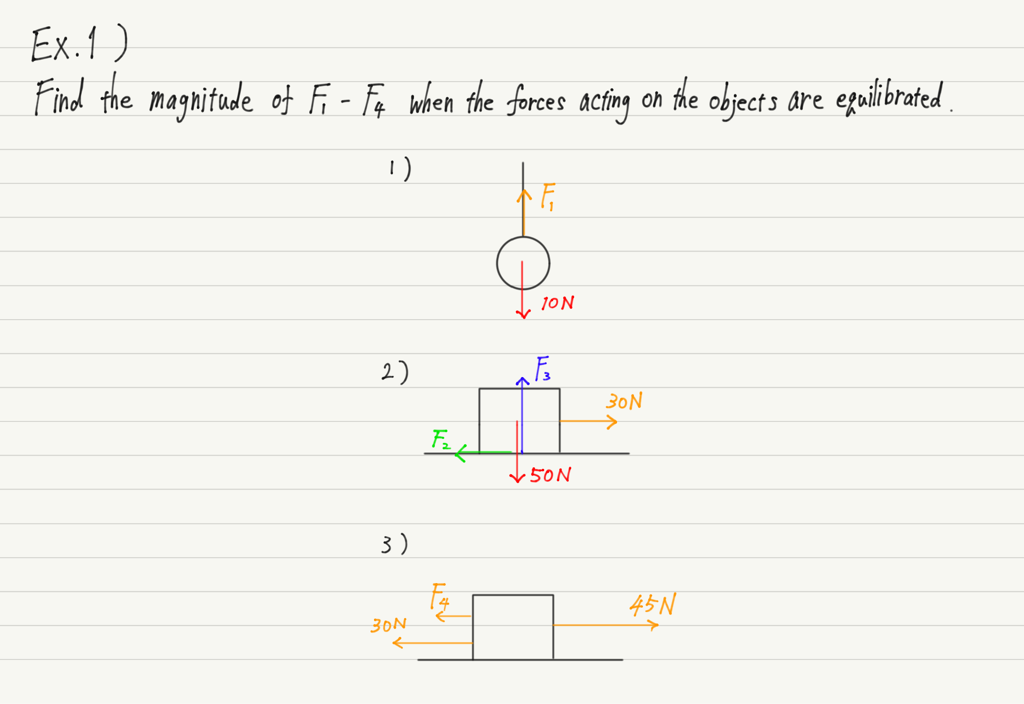Stop reading and do the calculation!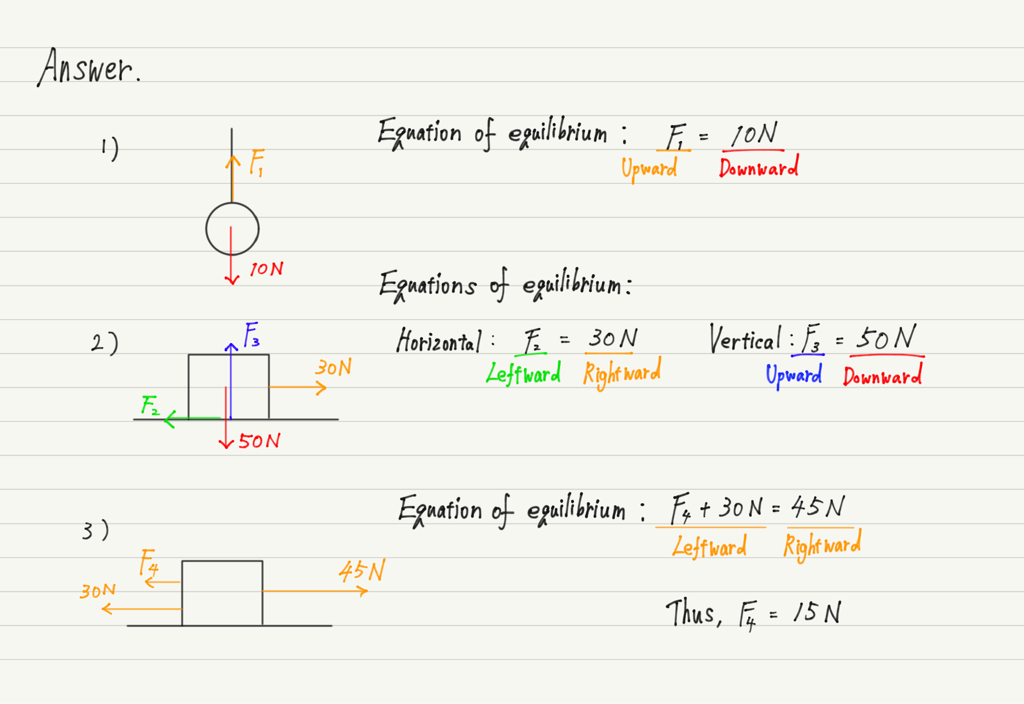Check the magnitude and direction of the force acting on the object. Then,

In the horizontal direction, “the magnitude of the rightward force = the magnitude of the leftward force”.

In the vertical direction, “the magnitude of the upward force = the magnitude of the downward force”.

The key is to formulate the equation like this!

When both horizontal and vertical forces are involved, as in 2), it is necessary to formulate the equation of equilibrium for each.

Let’s try one more exercise.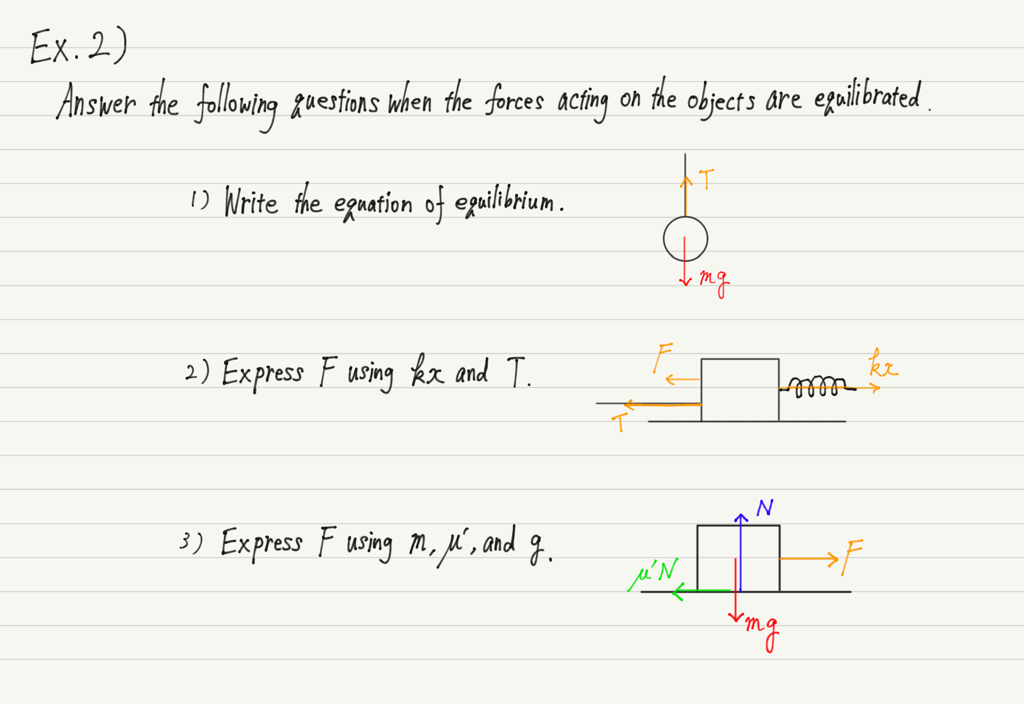Incidentally, mg is the magnitude of the gravitational force, kx is the elastic force, and μ’N is the expression for the kinetic friction.
(T and N are symbols for tension force and normal force, respectively.)

In the future, the forces that you have learned so far will appear frequently.
If you have forgotten them, please review them again.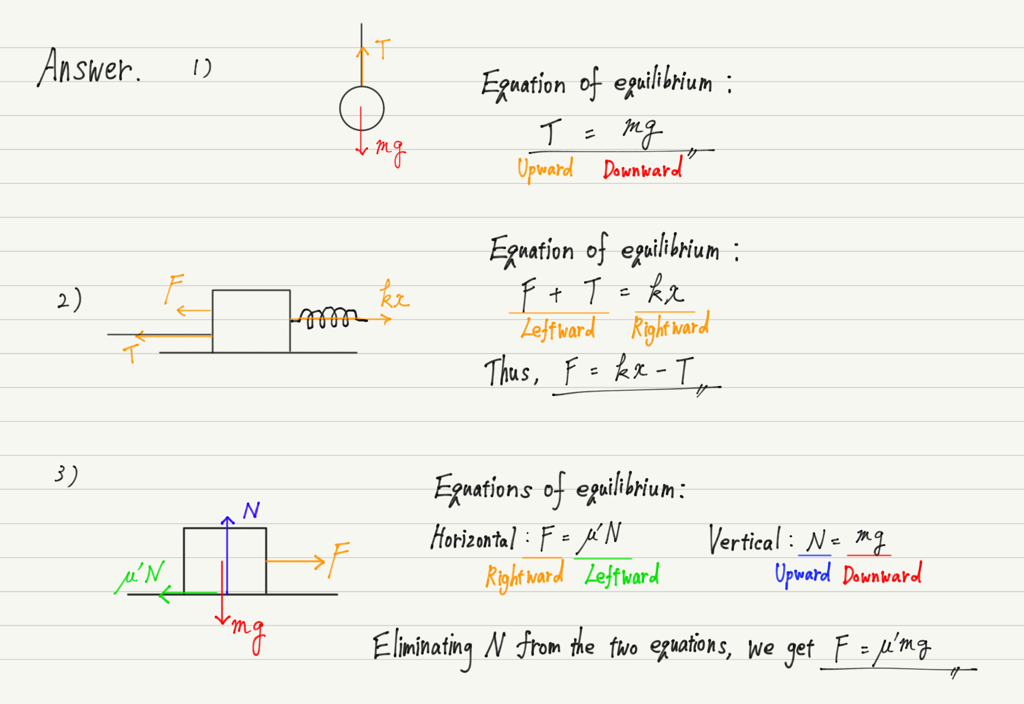The equation of equilibrium is very important in mechanics, so make sure you understand it perfectly.

## Summary of this lecture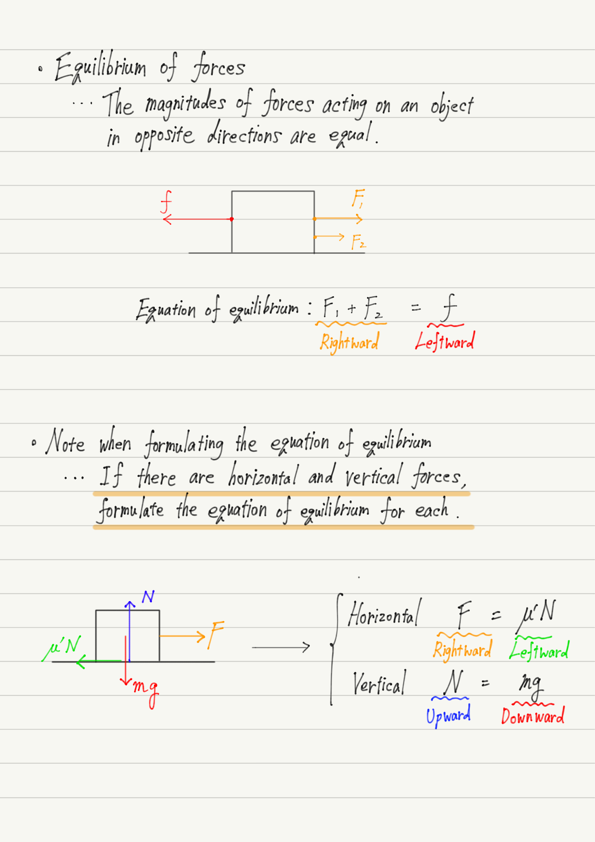## Next Time

In the next lecture, we will learn the laws of motion.

https://www.yukimura-physics.com/entry/dyn-f19

error: Content is protected !!